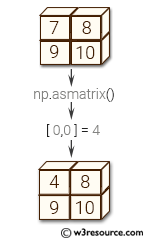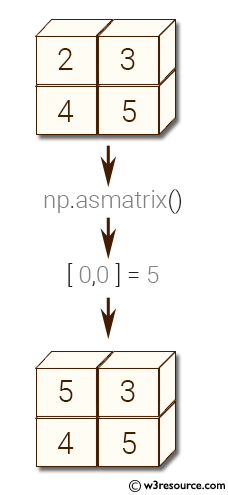# NumPy array creation: mat() function

## mat() function

The mat() function is used to interpret a given input as a matrix.
Unlike matrix, asmatrix does not make a copy if the input is already a matrix or an ndarray. Equivalent to matrix(data, copy=False).

Syntax:

`numpy.mat(data, dtype=None)`Version: 1.15.0

Name Discription Required / Optional
data input data. Required
dtype Data-type of the output matrix. optional

Return value:

mat : matrix - data interpreted as a matrix.

Example-1: NumPy.mat() method

``````>>> import numpy as np
>>> a = np.array([[2,3], [4,5]])
>>>
>>> y = np.asmatrix(a)
>>>
>>> a[0,0] = 5
>>>
>>> y
matrix([[5, 3],
[4, 5]])
``````

Pictorial Presentation:Python - NumPy Code Editor:

Previous: vander()
Next: bmat()

﻿